# 1 Thursday, August 18

Some examples of moduli spaces:

## 1.1 Picard varieties

Consider $$X=E$$ an elliptic curve, which can be defined as:

• A 1-dimensional abelian variety,
• A Weierstrass equation $$y^2 = x^3 + ax + b$$,
• A nonsingular genus 1 algebraic curve with a fixed origin, so $${\mathbf{C}}/\Gamma$$ for $$\Gamma\cong {\mathbf{Z}}^2$$ a lattice.

Recall that the group is \begin{align*} \operatorname{Pic}(X) \mathrel{\vcenter{:}}=\left\{{\text{Invertible } {\mathcal{O}}_X{\hbox{-}}\text{sheaves}}\right\}/\sim \cong \left\{{\text{Line bundles over } X}\right\}/\sim .\end{align*} There is a homomorphism $$\operatorname{Pic}(X) \xrightarrow{\deg} {\mathbf{Z}}\to 0$$ with $$\operatorname{Pic}^0(X) \mathrel{\vcenter{:}}=\ker \deg$$. A priori $$\operatorname{Pic}^0(X)$$ is a group, but in fact has the structure of a variety – there exists a Jacobian variety $$\operatorname{Jac}(X)$$ such that $$\operatorname{Pic}^0(X) \cong \operatorname{Jac}(C)(k)$$, the $$k{\hbox{-}}$$points of the Jacobian. Thus $$\operatorname{Jac}(X)$$ is a moduli space of invertible sheaves of degree zero.

For $$X=E$$ an elliptic curve, $$\operatorname{Jac}(E) \cong E$$.

There are distinct varieties with the same $$k{\hbox{-}}$$points: take for example the cuspidal curve $$X = V(y^2-x^3)$$ and $${\mathbf{A}}^1$$ – there is a map \begin{align*} {\mathbf{A}}^1 &\to X \\ t &\mapsto {\left[ {t^2, t^3} \right]} .\end{align*} with inverse $$t=y/x$$:Note that these have the same $$k{\hbox{-}}$$points over any field $$k$$. Thus we need to consider not just objects, but families of objects.

## 1.2 Elliptic curves

The moduli space of elliptic curves \begin{align*} {\mathcal{M}}_{1} = \left\{{\text{Elliptic curves over }{ \overline{k} }}\right\}_{/ {\cong}} .\end{align*} As an algebraic variety, $${\mathcal{M}}_1 \cong {\mathbf{A}}^1_j$$ (the $$j{\hbox{-}}$$line) coming from taking the $$j{\hbox{-}}$$invariant \begin{align*} j(X) = j(a, b) =_? {2a\over 4a^3 + 27b^2} .\end{align*}

Then if $$X\to S$$ is a family of genus 1 algebraic curves, there exists a unique map $$S\to {\mathbf{A}}^1_j$$ where $$s\in S$$ maps to $$j(X_s)$$. How would you prove this? See Hartshorne’s treatment using the Weierstrass $$\wp{\hbox{-}}$$function. Alternatively, factor to get $$y=x(x-1)(x-\lambda)$$ for $$\lambda\not\in\left\{{0, 1}\right\}$$ and quotient by $$S_3$$ acting by permuting $$\left\{{0,1,\lambda}\right\}$$. One can then form $$M_1 = {\mathbf{A}}^1_\lambda/S_3$$ and construct $$j(\lambda)$$ invariant under this action. Note that when $$X = \operatorname{Spec}R$$ is affine and $$G$$ is finite, there is an isomorphism $$\operatorname{Spec}R/G \cong \operatorname{Spec}R^G$$ to the GIT quotient. If $$X$$ is not affine but $$G$$ is finite, one can still patch together quotients locally.

## 1.3 Vector bundles

Moduli of sheaves or vector bundles (locally free $${\mathcal{O}}_X{\hbox{-}}$$modules of rank $$n$$) on a fixed base variety $$X$$, e.g. a curve. One might fix invariants like a rank $$r$$, degree $$d$$, etc in order to impose a finiteness/boundedness condition on the moduli space. For $$X = {\mathbf{P}}^1$$, a vector bundle $$F\to X$$ decomposes as $$F = \bigoplus _{i=1}^r {\mathcal{O}}(d_i)$$ where $$\deg F = \sum d_i$$ by Grothendieck’s theorem. Since some $$d_i$$ can be negative, the moduli come in a countably infinite set. To impose boundedness one can additionally add stability conditions such as semistability, which here ensures only finitely many degrees appear and the existence of a moduli space $${\mathcal{M}}_{r, d}(X)$$. To do this, twist by a large integer and take global sections to get $$H^0(X; F(n))$$ for $$n\gg 0$$. Understanding $$\bigoplus_{n\geq 0} H^0(X; F(n))$$ as a module over $$R = \bigoplus _{n\geq 0} {\mathcal{O}}(n)$$ allows one to reconstruct $$F$$. Thus one can construct $${\mathcal{M}}_{r, d}(X) = ? / \operatorname{PGL}_N$$ corresponding to choosing a basis for $$H^0$$. Here we remove some “unstable” locus before taking the quotient – note that points correspond to orbits, except that some orbits become identified.

This is an “easy” moduli problem, since vector bundles are somehow linear. See Ramanujan, ?, Mumford, 40+ years ago.

## 1.4 Nonlinear examples: moduli of curves/varieties

Let $${\mathcal{M}}_2$$ be the moduli of curves $$C$$ with $$g(C) = 2$$. All such curves are hyperelliptic, so similar to the $$g=1$$ theory. In the $$g=1$$ case, curves can be realized as ramified covers of $${\mathbf{P}}^1$$: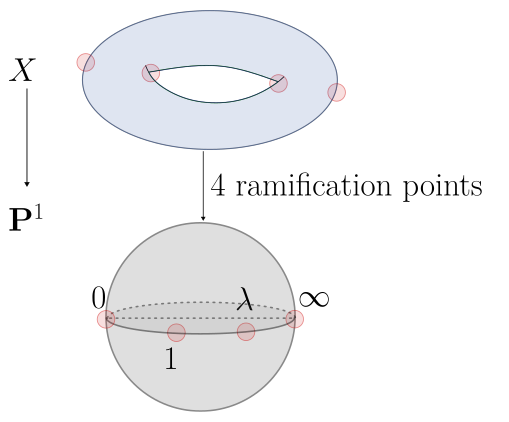In the $$g=2$$ case, they can similarly be realized as 2-to-1 maps ramified at 6 points: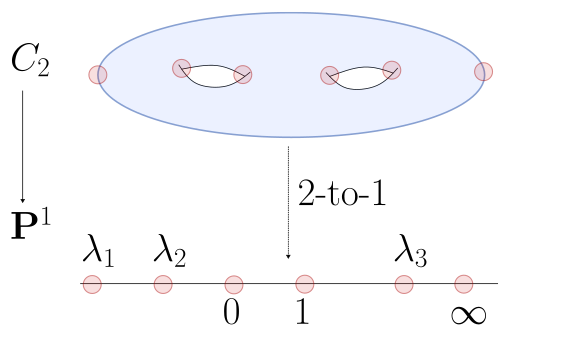One can realize $${\mathbf{A}}^3\supseteq U \mathrel{\vcenter{:}}=\left\{{{\left[ { \lambda_1, \lambda_2, \lambda_3} \right]} {~\mathrel{\Big\vert}~}\lambda_i\neq 0,1,\infty, \lambda_i \neq \lambda_j}\right\}$$ and $$M_2 = U/S_6$$.

For $$g=3$$, one has $$g=(1/2)(d-1)(d-2)$$ by the adjunction formula, so $$g=3$$ corresponds to $$d=4$$ and one obtains

• Hyperelliptic: degree 4 curves in $${\mathbf{P}}^2$$ (the generic case), or

• Non-hyperelliptic: 2-to-1 covers of $${\mathbf{P}}^1$$ ramified at 8 points.

There is no analog of the Weierstrass equation for degree 4 polynomials, so write $$f_4(x_1, x_2, x_3) = \sum a_m x^m$$ where $$x^m \mathrel{\vcenter{:}}= x_1^{m_1}x_2^{m_2}x_3^{m_3}$$. How many such polynomials are there? Count points in the triangle: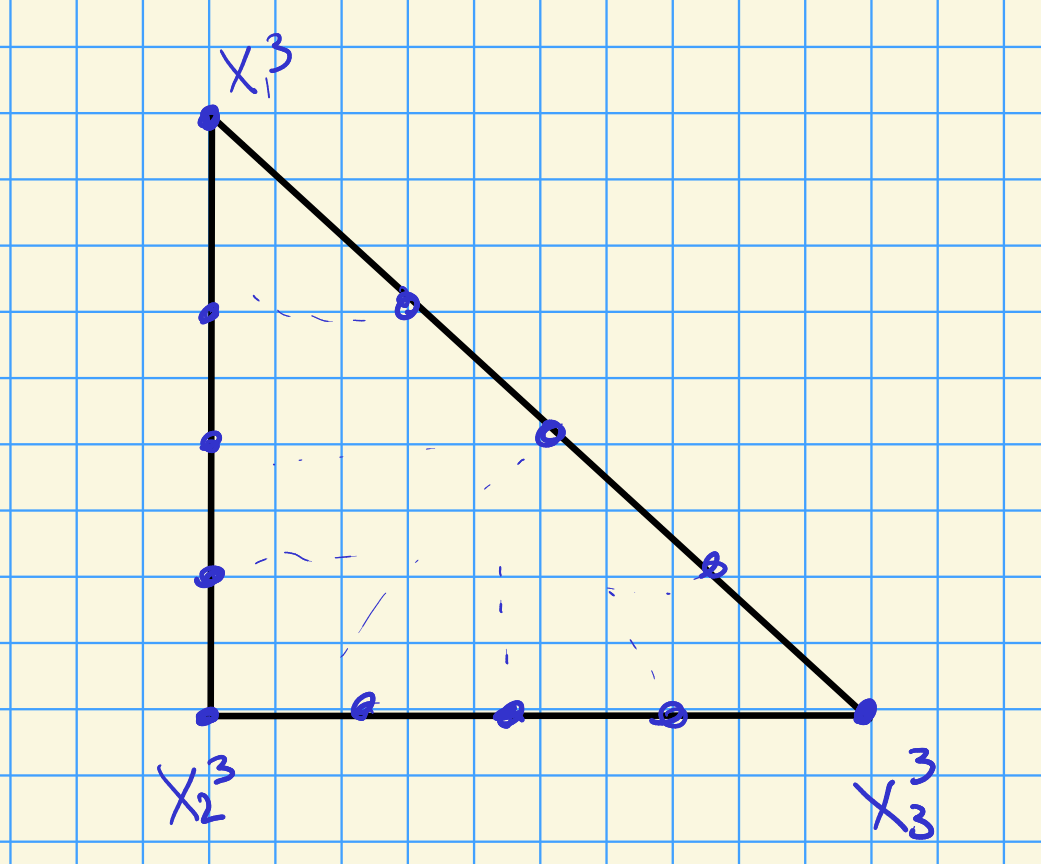This yields $$5+4+3+2+1 = 15$$ such monomials, and one can write \begin{align*} {\mathbf{A}}^{15}\setminus\left\{{0}\right\}/ k^{\times}= {\mathbf{P}}^{14} \supseteq U = {\mathbf{P}}^{14}\setminus\Delta \end{align*} where $$\Delta$$ is the discriminant locus. This is an affine variety, since $$\Delta$$ is a high degree hypersurface. Then form $$U/\operatorname{PGL}_3$$, noting that $$\dim \operatorname{PGL}_3 = 3^2-1 = 8$$, so $$\dim {\mathbf{P}}^{14}\setminus\Delta = 14-8 = 6$$.

Ways of forming moduli spaces:

• GIT,
• Hodge theory over $${\mathbf{C}}$$,
• Stacks (e.g. Artin’s method).

These rarely produce compact/complete spaces, so we’ll discuss compactification. Why compactify? Computing things, projectivizing, intersection theory. See Bailey-Borel and toroidal compactifications.

A note on Hodge theory: for an elliptic curve, one can write $$E = {\mathbf{C}}/\left\langle{1, \tau}\right\rangle$$ with $$\Im(\tau) > 0$$ (so $$\tau\in {\mathbb{H}}$$), one can form $${\mathcal{M}}_1 = \dcosetr{{\mathbb{H}}}{{\operatorname{SL}}_2({\mathbf{Z}})}$$. This is Hodge theory: $$\tau$$ is a period, and we quotient a bounded symmetric domain by an arithmetic group. Similarly, for PPAVs one can write $${\mathcal{A}}_g = \dcosetr{H_g}{{\mathsf{Sp}}_{2g}({\mathbf{Z}})}$$, and for K3 surfaces one has $$F_{2d} = {\mathbb{D}}_{2g}/\Gamma_{2g}$$ where $$\omega_X \in {\mathbb{D}}$$. One can determine things like Jacobians using Torelli theorems.

Todo: how much do you know, and what are you trying to get out of the course?

# 2 Tuesday, August 23

See Mukai’s book

## 2.1 An aside on branched covers of curves

A question from me: is every curve a branched cover of $${\mathbf{P}}^1$$ over some number of points? Consider maps $$f: X\to {\mathbf{P}}^1$$ where $$f(X) \not\subset {\mathbf{P}}^{n-1}$$ is not contained in a hyperplane. This biject with basepoint free linear systems – let $${\mathcal{F}}$$ be an invertible sheaf, then a linear system is any linear subspace $$V \subseteq H^0(X; {\mathcal{F}})$$. Writing $$V = \left\langle{f_i}\right\rangle_{i=0}^n$$, the bijection is sending $$p\mapsto {\left[ {f_0(p): \cdots : f_n(p)} \right]} \in {\mathbf{P}}^{n}$$. Since $${\mathcal{F}}$$ is invertible, locally $${ \left.{{{\mathcal{F}}}} \right|_{{U}} } \cong {\mathcal{O}}_U$$ – this map is well-defined precisely when not all the $$f_i(p)$$ are zero, which is precisely the basepoint-free condition. A map $$f:X\to {\mathbf{P}}^1$$ thus corresponds to two sections which don’t simultaneously vanish. If $$X$$ is projective, it admits a very ample line bundle $${\mathcal{L}}$$ where the base locus of $$H^0({\mathcal{L}})$$ is empty. One can now project away from any point outside of the curve to get a regular map factoring the projective embedding:The projection: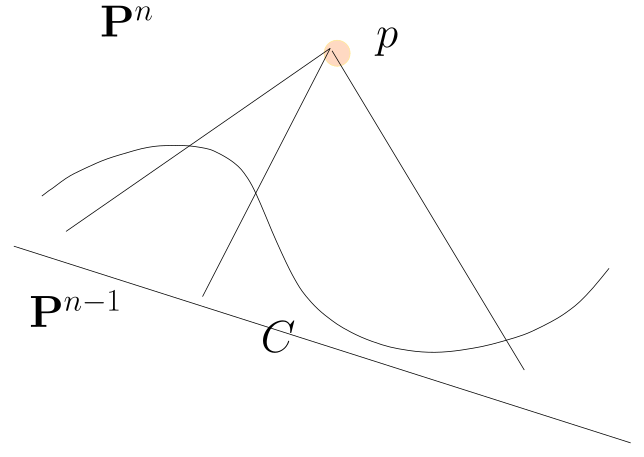One can continue to project until reaching $${\mathbf{P}}^1$$.

The gonality of a curve $$X$$ is the minimal degree of a map $$X\to {\mathbf{P}}^1$$, where degree is the size of a generic fiber. Here a cover may have a ramification locus upstairs and a branch locus downstairs, which are small in the sense that they are algebraic subsets.

Recall $$X$$ is hyperelliptic if it admits a 2-to-1 map $$X\to {\mathbf{P}}^1$$, so has gonality 2. Gonality 1 curves are isomorphic to $${\mathbf{P}}^1$$, and gonality 3 are trigonal.

## 2.2 Course plan

Plan for the course:

1. GIT ($$1/2$$ to $$2/3$$ of the course). Goals:
• Construct moduli of vector bundles on a curve, surface, etc.
• Construct $${\mathcal{M}_g}$$ (Riemann’s moduli space, not complete, projective, affine, but quasiprojective), $$\overline{{\mathcal{M}_g}}$$ (Deligne-Mumford’s moduli of stable curves), $$\overline{{\mathcal{M}}}_{0,n}$$, and $$\overline{{ \mathcal{M}_{g, n} }}(V)$$ (Kontsevich’s moduli of stable maps, to rigorously define Gromov-Witten invariants).1
• Sources:
• Mukai’s book, An Introduction to Invariants and Moduli (this will be our primary source). He covers stable vector bundles on curves.
• Mumford’s book on GIT and his paper about stability for algebraic curves, although this is perhaps unnecessarily difficult! We’ll need this for $$\overline{{\mathcal{M}_g}}$$.
1. Hodge theoretic approaches. Goals:
• Construct $${\mathcal{A}_g}$$ the moduli of abelian varieties. See Birkenhake and Lange.
• Construct $$F_{2d}$$ the moduli of K3 surfaces. See Huybrechts.

## 2.3 Intro

Let $$X$$ be a genus $$g$$ smooth projective curve. Over $${\mathbf{C}}$$, projective implies compact, and non-projective is a Riemann surface with finitely many punctures. More generally, over $$k={ \overline{k} }$$ smooth means that $$\dim {\mathbf{T}}_{X, x} = \dim X$$ at every point $$x\in X$$. Note that $$X^{{\mathrm{sing}}} \subseteq X$$ is an algebraic and thus closed subset, so curves have finitely many singularities (nodes, cusps, etc). There is only one topological type of curve, but there are distinct algebraic and conformal structures (which turn out to be equivalent notions for curves).

One can show $${\mathcal{M}}_g({\mathbf{C}})$$ is an orbifold of dimension $$3g-3$$, i.e. locally a quotient $$M/G$$ of a manifold by a finite group. Similarly $${\mathcal{M}}_g$$ is a quasiprojective algebraic variety of dimension $$3g-3$$ with only quotient singularities. Mumford was the first to ask questions about its geometry, e.g. is it rational?

A variety $$X\in \mathsf{Alg}{\mathsf{Var}}_{/ {k}}$$ is rational if $$X\overset{\sim}{\dashrightarrow}{\mathbf{P}}^n$$, so there is a common open subset $$X\supseteq U \subset {\mathbf{P}}^n$$.2 Equivalently, there is an isomorphism of rational functions \begin{align*} k(X) \cong k({\mathbf{P}}^n)\cong k({\mathbf{A}}^n) ,\end{align*} where the latter is comprised of quotients of polynomials. One can take $$n=\dim X$$, since if $$N > n$$ one can factor a dominant map $${\mathbf{P}}^N \to X$$ through a hyperplane $${\mathbf{P}}^{N-1}\to X$$ which is still dominant.

$$X$$ is unirational if there is a dominant morphism $$f: {\mathbf{P}}^n \overset{\sim}{\dashrightarrow}X$$, so a map defined on an open subset whose image is dense. Equivalently, $$X$$ admits a parameterization by coordinates $$x_1, \cdots, x_n$$, so there is a rational parameterization.3

In this case, there is a degree $$d$$ finite extension $$k(x_1,\cdots, x_n)$$ over the pullback of $$k(X)$$.

Is the converse true? I.e. if there is a finite extension $$k(x_1,\cdots, x_n)$$ over $$k(X)$$, is it true that $$k(X) = k(y_1,\cdots, y_n)$$? So does unirational imply rational?

Lüroth proved this in dimension 1, and as a consequence of the classification of surfaces, the Italian school showed this in dimension 2. See the Castelnuovo criterion, which shows $$X$$ is rational iff $$X$$ is regular, i.e. $$q \mathrel{\vcenter{:}}= h^1(X; {\mathcal{O}}_X) = 0$$ and $$p_2 \mathrel{\vcenter{:}}= h^0(X; 2K_X) = 0$$.

This is false in dimension 3. 3-4 counterexamples were given in the 70s/80s, first due to Iskovskih-Manin, a second due to Clemens-Griffith, and later due to Mumford.

Show that if $$k \subsetneq K \subset k(X)$$, then $$K$$ is monogenic (generated by a single element).

$${\mathcal{M}_g}$$ is rational for $$g=2$$.

Note that $$3(g-1)=3$$, and a genus 2 curve is a branched cover $$X\to {\mathbf{P}}^1$$ ramified at 6 points $$\left\{{0,1,\infty, \lambda_1, \lambda_2, \lambda_3}\right\}$$. This yields a dominant map $${\mathbf{A}}^3\to {\mathcal{M}}_2$$ which is finite-to-1 and defined up to the action of $$S_6$$. This is not defined if points collide, which corresponds to collapsing cycles in $$X$$, and is degree $$6!$$. Here we can write $$X = V(y^2 = x(x-1)(x - \lambda_1) (x- \lambda_2)(x - \lambda_3)) \subseteq {\mathbf{A}}^2_{/ {{\mathbf{C}}}}$$. If any $$\lambda_i = \lambda_j$$ for $$i\neq j$$, one obtains a singularity locally modeled on the node $$y^2=x^2$$, which is the following over $${\mathbf{R}}$$: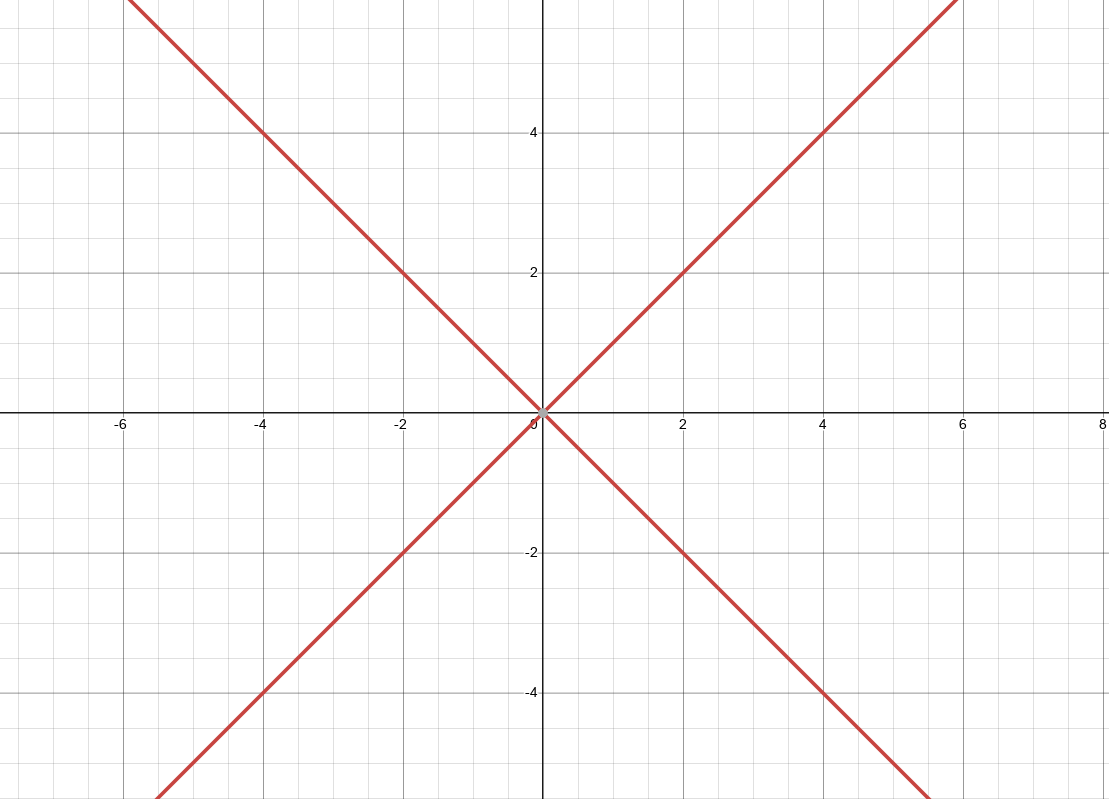Over $${\mathbf{C}}$$, this is two hyperplanes intersecting in a single point. We can thus write \begin{align*} {\mathcal{M}}_2 = {\mathbf{A}}^3\setminus\left\{{ \lambda_i = \lambda_j {~\mathrel{\Big\vert}~}i\neq j }\right\} / S^6 ,\end{align*} which is rational and unirational.

$${\mathcal{M}}_3$$ is rational and unirational.

We need to show that a genus 3 curve can be parameterized by 6 parameters. Noting that a genus 3 curve is planar of degree 4, which suffices – planar curves are given by polynomials $$f_4(x_1, x_2, x_3) = \sum a_n x^n$$, and these are the parameters.

One of the classical Italian algebraic geometers (either Severi or Castelnuovo) “proved” the false statement that $${\mathcal{M}_g}$$ is unirational for all $$g$$. In fact this is only true for $$g\leq 9$$. The idea is good though: any curve $$X\hookrightarrow{\mathbf{P}}^n$$ can be projected to a curve in $$X \to {\mathbf{P}}^2$$ with only finitely many nodes. The coordinates for the nodes can serve as parameters. Having a curve pass through given points is a linear condition, as is saying it is singular at a point (by computing partial derivatives). Being a node is not a linear condition – instead, it is a quadratic algebraic condition coming from the vanishing of a $$2\times 2$$ determinant. It’s also not clear that imposing singularity conditions locally are all independent, since singularities at some points can force singularities at others. Mumford proved that $${\mathcal{M}_g}$$ is not unirational for $$g\geq 24$$, and is in fact general type, which is far from unirational.

Next time: more general introduction, stable curves, a bit about Hodge theory, then starting Mukai’s book.

# 3 Thursday, August 25

Goal: showing $${\mathcal{M}_g}$$ exists as a quasiprojective complex variety, and can in fact be defined over any field $$k$$ or even over $${\mathbf{Z}}$$. Here quasiprojective over $${\mathbf{Z}}$$ means $$X \subseteq {\mathbf{P}}^n_{/ {{\mathbf{Z}}}}$$ is a closed subset given as $$X = V(f_i)$$ for homogeneous integral polynomials $$f_i$$. Note that $${\mathcal{M}_g}({ \overline{k} }) = \left\{{\text{smooth projective curves of genus } g}\right\} = X({ \overline{k} }) \setminus Z({ \overline{k} }) \subseteq {\mathbf{P}}^n_{/ {{ \overline{k} }}}{_{/ \sim} }$$ where $$Z = V(f_i, g_i)$$ – this says $${\mathcal{M}_g}$$ satisfies exactly the equations $$f_i$$ and no more. Anytime objects have isomorphisms, one only gets a coarse moduli space instead of a fine moduli space, which we’ll later describe. Families $${\mathcal{X}}\to S$$ yield to maps $$S\to {\mathcal{M}_g}$$ over $$\operatorname{Spec}{ \overline{k} }$$, and this will be a bijection when $${\mathcal{M}_g}$$ is a fine moduli space and $${\mathcal{X}}$$ is the pullback of a universal family $${\mathcal{E}}\to{\mathcal{M}_g}$$. Since we only have a coarse moduli space, a family yields a map to $${\mathcal{M}_g}$$, but these are not in bijection.

We’ll want projective varieties in order to do intersection theory. The most fundamental compactification: the Deligne-Mumford compactification $$\overline{{\mathcal{M}_g}}$$ of $${\mathcal{M}_g}$$, i.e. the moduli of stable curves of genus $$g$$. This is a projective moduli space containing $${\mathcal{M}_g}$$ as an open dense subset, and is obtained by adding degenerate curves “at infinity.”

Consider $$x_0 x_2 = t^n x_1^2$$ in $${\mathbf{P}}^2_{x_0, x_1, x_2}$$ and take the 1-parameter degeneration $$t\to 0$$. This is smooth for $$t\neq 0$$, since this is a full rank conic. In affine coordinates this is $$xy=t^n$$, which degenerates to the simple node (double point) $$xy=0$$. Part of this degeneration data can be recovered from a tropical curve, which is a metric graph whose points are singularities and lengths correspond to the $$n$$ in $$t^n$$: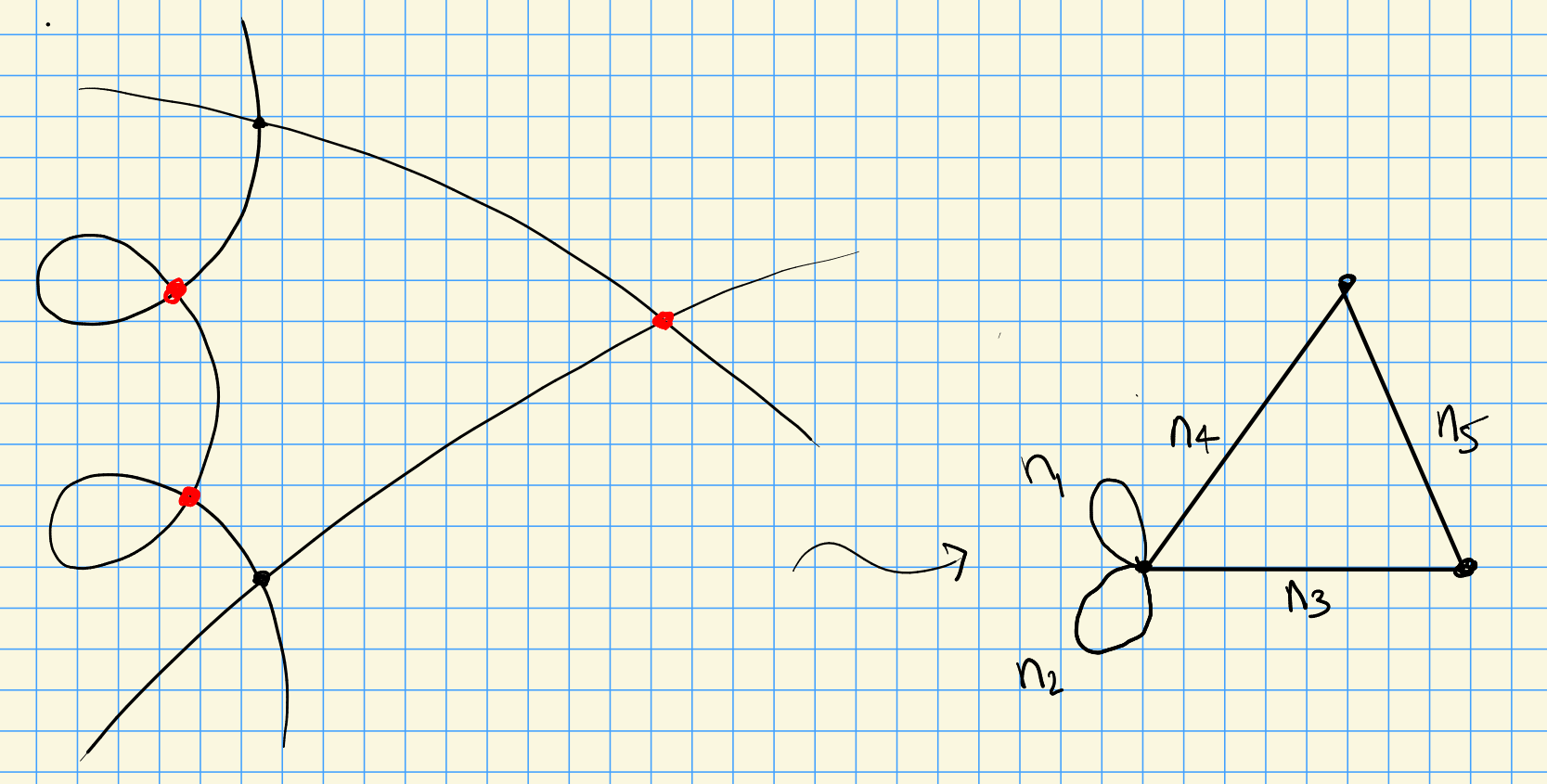A stable curve of genus $$g$$ is a connected reduced (possibly reducible) projective curve $$C$$ such that

• (Mild singularities) $$C$$ has at worst nodes, locally of the form $$xy=0$$.
• (Numerical) The dualizing sheaf $$\omega_X$$ is ample.

Note that $$g=h^0(\omega_X) = h^1({\mathcal{O}}_X)$$.

Writing a multi-component curve as $$X = \bigcup X_i$$, the numerical condition requires that for every $$X_i\cong {\mathbf{P}}^1$$, one has $${\left\lvert {X_i \cap(X\setminus X_i)} \right\rvert} \geq 3$$, and for all $$X_i$$ of the following form (or $$X_i\cong E$$ an elliptic curve), $${\left\lvert {X_i \cap(X\setminus X_i)} \right\rvert} \geq 1$$:This is equivalent to $${\sharp}\mathop{\mathrm{Aut}}X < \infty$$. For $$g\geq 2$$ and $$C_g$$ smooth of genus $$g$$, one has $${\sharp}\mathop{\mathrm{Aut}}C_g < \infty$$, and for $$g=1$$ enforces $$\dim \mathop{\mathrm{Aut}}C_g = 1$$. For $$g=0$$, note $$\mathop{\mathrm{Aut}}{\mathbf{P}}^1 = \operatorname{PGL}_2$$ which has dimension 3, so fixing at least 3 points cuts this down to a finite automorphism group.

The dualizing sheaf $$\omega_X$$ is invertible if $$X$$ has only nodes. The adjunction formula yields a twist $${ \left.{{\omega_X}} \right|_{{X_i}} } = \omega_{X_i}(X\setminus X_i)$$ Then $$\omega_X$$ is ample iff $$\deg{ \left.{{\omega_X}} \right|_{{X_i}} } > 0$$. One can compute $$\deg \omega_{X_i}(X\setminus X_i) = 2g_i - 2 + {\left\lvert {X_i \cap(X\setminus X_i)} \right\rvert}$$, hence the lower bound on the number of intersection points.

Without the numerical condition, the limit is not unique.

To see this, take a trivial family over $${\mathbf{P}}^1$$, so a surface, and blow up a point on the central fiber. This yields a multi-component curve, which we allow, and we can continue blowing up such points: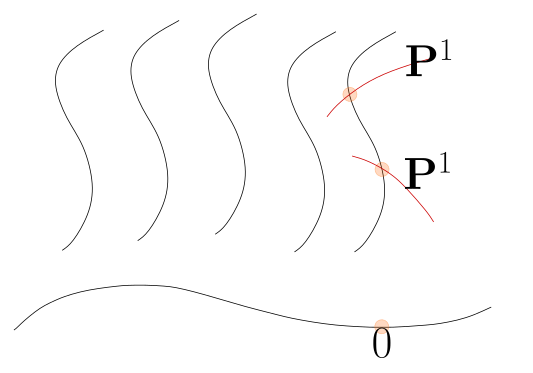If $$\omega_{X_t}$$ is ample for all $$t$$, then $$\omega_{{\mathcal{X}}}/S$$ is relatively ample, which implies $${\mathcal{X}}/S$$ is the canonical model. One can contract $$(-1)$$ curves to get a minimal model, and $$(-2)$$ curves to get canonical models. See degenerations of elliptic curves to wheels of copies of $${\mathbf{P}}^1$$:See Kodaira’s elliptic fibers – classified by extended Dynkin diagrams $$\tilde A_n, \tilde D_n, \tilde E_n$$, and special types $$\tilde A_i^*$$ for $$i=0,1,2$$: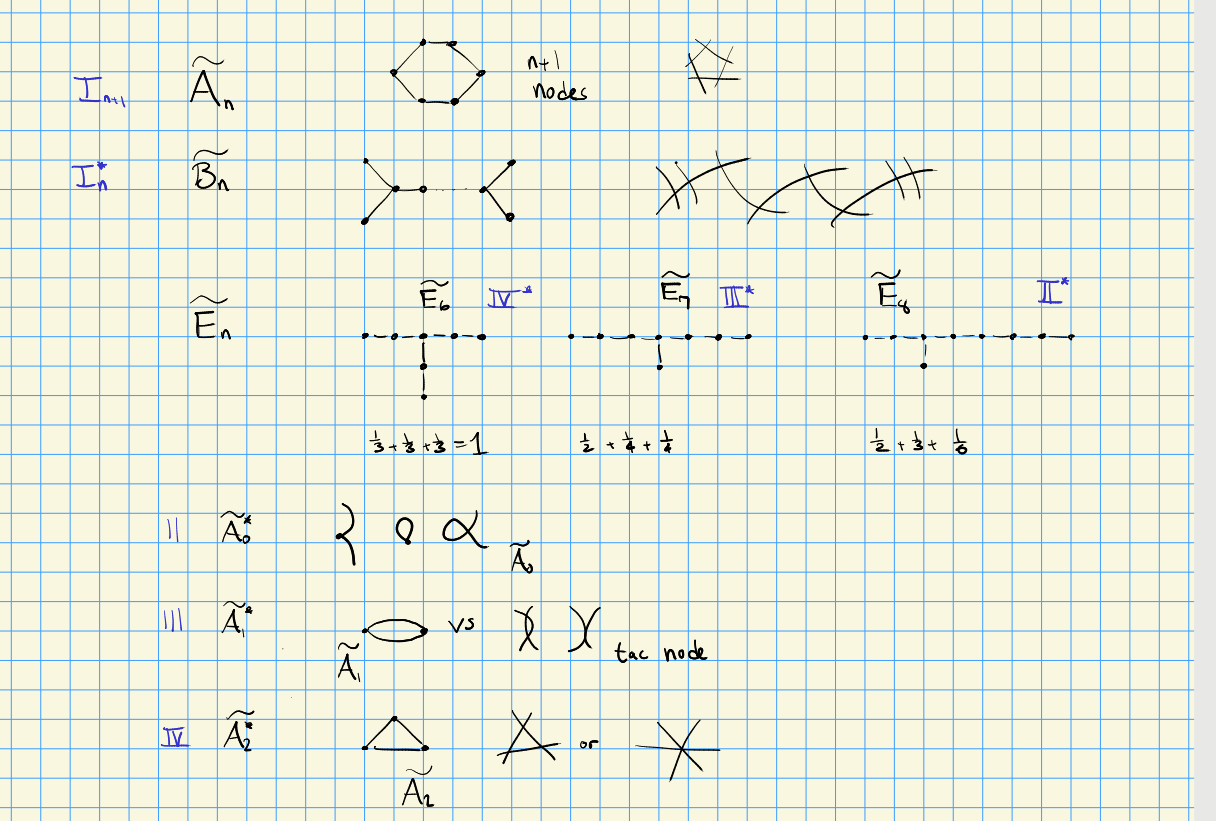For $$V$$ a projective variety, a stable curve is a map $$f:C\to V$$ satisfying

• (Mild singularities) $$C$$ has at worst nodes.
• (Numerical condition) $$\omega_C$$ is very ample, or equivalently has positive degree on components which map to points.

So for example, we can ignore vertical curves: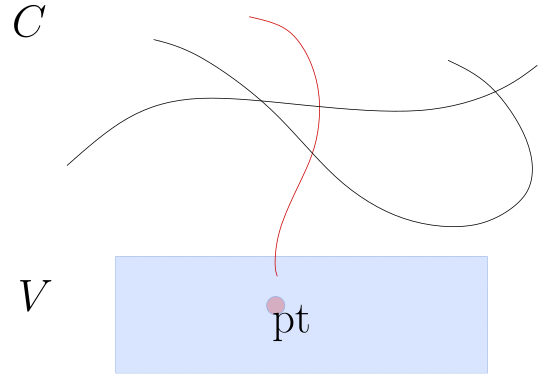One can define a moduli space of stable curves passing through $$n$$ marked points, $$\overline{{\mathcal{M}}}_{g, n}(V)$$: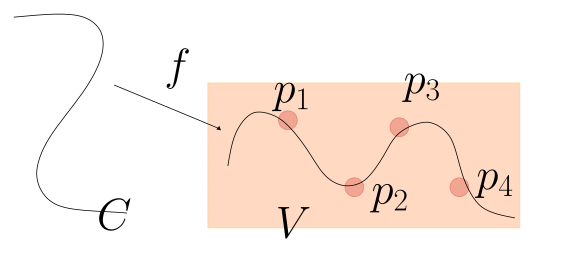Defined to formulate Gromov-Witten invariants. Motivated by physics, but originally non-algebraic and used almost complex structures. The second condition yields unique limits since it will yield a relative canonical model, which exist and are unique. This moduli space can be generalized to higher dimensions, see KSBA compactifications.

Constructing $${\mathcal{M}_g}$$ and $$\overline{{\mathcal{M}_g}}$$:

• Step 1: Parameterize embedded curves $$C_g \hookrightarrow{\mathbf{P}}^N$$ by the picking a basis of the linear system $${\left\lvert {2K_X} \right\rvert}$$, where $$N = 2(2g-2) - (g-1) - 1 = 3g-4$$ and $$\deg C_g = 2(2g-2)$$. Use either the Chow variety $$\mathsf{Ch}_{d, N}$$, parameterizing cycles/subvarieties of $${\mathbf{P}}^N$$ with degree $$d$$, or the Hilbert scheme $$\operatorname{Hilb}_h$$ parameterizing closed subschemes $$X \hookrightarrow{\mathbf{P}}^N$$ with a fixed Hilbert polynomial $$h$$. The latter may not yield reduced curves, but closed subschemes are easier than varieties since they are just defined by equations.

• Step 2: Divide by $$\operatorname{PGL}_{N+1}$$, using GIT (next week) to produce a space $$X/G$$ whose points (ideally) correspond to $$G{\hbox{-}}$$orbits.

# 4 Tuesday, August 30

Goal: understanding quotients of varieties by general group actions, a basic notion for moduli. The easiest case: finite groups $$G\curvearrowright X\in{\mathsf{Aff}} \mathsf{Alg}{\mathsf{Var}}_{/ {k}}$$ for $$k={ \overline{k} }$$.

Think of $$X \subseteq {\mathbf{A}}^n_{/ {k}}$$ for some $$N$$, with coordinates $${\left[ {a_1,\cdots, a_n} \right]}$$, so $$X = V(f_1,\cdots, f_n)$$. Note $${\mathcal{O}}_{{\mathbf{A}}^n} = k[x_1, \cdots, x_{n}]$$, and regular functions on $$X$$ are restricted polynomials, so we get a sequence \begin{align*} R = k[X] \leftarrow k[x_1, \cdots, x_{n}]\leftarrow I = \sqrt{\left\langle{f_1,\cdots, f_n}\right\rangle} ,\end{align*} so $$R\in {}_{k} \mathsf{Alg}^{\mathrm{fg}}$$ without nilpotents – in fact varieties biject with such algebras. If $$G\curvearrowright R$$ any ring, one can take invariants \begin{align*} R^G \mathrel{\vcenter{:}}=\left\{{r\in R {~\mathrel{\Big\vert}~}g^*(r) = r\,\,\forall g\in G}\right\} \end{align*} which is a subring and a $$k{\hbox{-}}$$subalgebra of $$R$$. Here $$g^*$$ is defined in terms of pullbacks of functions:If $${\sharp}G\notdivides \operatorname{ch}(k)$$ then $$R^G\in {}_{k} \mathsf{Alg}^{{\mathrm{fg}}}$$.4

There is a $$k{\hbox{-}}$$linear averaging map \begin{align*} S: R &\to R^G \\ r &\mapsto {1\over {\sharp}G} \sum_{g\in G} g^*(r) ,\end{align*} noting that $$S$$ is not a ring morphism.

Let $$a\in R$$ and consider $$p_a(x) \mathrel{\vcenter{:}}=\prod_{g\in G} (x - g^*(a) )$$, a polynomial of degree $$n = {\sharp}G$$ whose coefficients are in the subring $$R^G$$ and are symmetric polynomials in the $$g^*(a)$$. Since $$p_a(a) = 0 = a^n + \cdots$$, $$a^n$$ is a linear combination of $$1,a,\cdots, a^{n-1}]$$ with coefficients in $$R^G$$ and these symmetric polynomials. So if $$\left\{{a_1,\cdots, a_m}\right\}$$ generate $$R$$ as a $$k{\hbox{-}}$$algebra, the images of monomials $$S(a_1^{k_1}\cdots a_m^{k_m})$$ with $$0 \leq k_i \leq n$$ generate $$R^G$$. If $$b\in R^G$$, one one hand $$b = S(b)$$, and on the other hand $$b = \sum c_k a_I^{k_I}$$ so $$S(b) = \sum c_k S(a_I^{k_I})$$. Thus the $$c_k$$ are in the subring generated by elementary symmetric polynomials in the $$g^*(a_i)$$.

There is another basis for elementary symmetric polynomials given by Newton sums. Recall

• $$\sigma_1 = \sum x_i$$
• $$\sigma_2 = \sum x_i x_j$$
• $$\sigma_n = \prod x_i$$

The Newton sums are

• $$N_1 = \sum x_i$$
• $$N_2 = \sum x_i^2$$
• $$N_n = \sum x_i^n$$,

and one can inductively show that one can be written in terms of the other.

The advantage is that the averaging operator commutes with sums, so the $$c_k$$ like in the subring generated by Newton sums of the $$S(a_i^{k_i})$$

Assume $$G$$ is finite and acts on $$X\in{\mathsf{Aff}} \mathsf{Alg}{\mathsf{Var}}_{/ {k}}$$. There is a bijection \begin{align*} \left\{{G{\hbox{-}}\text{orbits on } X}\right\} \rightleftharpoons\left\{{\text{Points of an affine variety $Y$ with } k[Y] = k[X]^G}\right\} .\end{align*} Writing $$X = \operatorname{mSpec}R$$ (since we’re working with varieties over a field), one can write $$Y = \operatorname{mSpec}(R^G)$$. There is a quotient map $$\pi: X\to Y$$ which is universal with respect to maps $$G{\hbox{-}}$$equivariant maps $$\psi: X\to Z$$ with $$Z$$ affine.5 This gives a geometric and a categorical quotient.

Since $$R^G \hookrightarrow R$$ we obtain a morphism $$X \xrightarrow{\pi} Y$$ of varieties and a pullback $$k[Y] \xrightarrow{\pi^*} k[X]$$. Given $$\phi\in k[Y]$$, the pullback $$\pi^*( \varphi)$$ is constant on $$G{\hbox{-}}$$orbits. Given two orbits $$O_1, O_2$$, one can find an invariant function which is zero on $$O_1$$ and one on $$O_2$$. Any finite subset on a variety is closed. Delete a point from $$O_2$$ to get a proper containment of sets $$O_1 \cup(O_2\setminus\left\{{ p }\right\}) \subset O_1 \cup O_2$$ which are both closed in $$X$$. This corresponds to a proper containment of ideals, so pick a function vanishing on the former but not the latter and average. Thus the regular invariant functions separate orbits, and the images of the $$O_i$$ in $$Y$$ are distinct, making $$X\to Y$$ a geometric quotient.

For the universal property, any $$X\to Z$$ defines a ring morphism $$S\to R$$, and $$G{\hbox{-}}$$equivariance factors this as $$S\to R^G \hookrightarrow R$$, thus factoring $$X\to Y\to Z$$.

The right classes of groups to take: geometrically reductive and linearly reductive.6

Over $${\mathbf{C}}$$ these coincide, and are e.g. $$\operatorname{GL}_n({\mathbf{C}})$$ (trivial center, nontrivial $$\pi_1$$), the classical semisimples

• Type A, $${\operatorname{SL}}_n({\mathbf{C}})$$ (nontrivial center, trivial $$\pi_1$$),
• Types B and D, $${\operatorname{SO}}_{n}({\mathbf{C}})$$,
• Type C $${\operatorname{Sp}}_{2n}({\mathbf{C}})$$,

along with $$({\mathbf{C}}^{\times})^n$$, and their products and extensions, and the exceptional groups $$E_6, E_7, E_8, F_4, G_2$$. Here linearly reductive means any finite-dimensional representation decomposes into a sum of irreducible representations.

The most useful for moduli: $$\operatorname{GL}_n, \operatorname{PGL}_n, {\operatorname{SL}}_n$$. Note that $$\operatorname{PGL}$$ and $${\operatorname{SL}}$$ are almost the same, up to a finite group.

For $$\operatorname{ch}(k) = p$$, the only linearly reductive group on this list is $${\mathbf{G}}_m^n$$, while “geometrically reductive” includes all of these groups. Over $${\mathbf{Z}}$$, the split versions $$\operatorname{GL}_n({\mathbf{Z}}), {\operatorname{Sp}}_n({\mathbf{Z}})$$, etc still work.

Nonsplit groups are e.g. those not isomorphic to $$\operatorname{GL}_n(k)$$ but become isomorphic over $${ \overline{k} }$$. Examples: compare $${\mathbf{G}}_m$$ over $${\mathbf{R}}$$ and $$S^1 = k[x,y]/\left\langle{x^2+y^2-1}\right\rangle$$; these only become isomorphic over $${\mathbf{C}}$$.

Let $$S_n\curvearrowright k[x_1, \cdots, x_{n}]$$ by permuting variables, then \begin{align*} k[x_1, \cdots, x_{n}]^{S_n} = k[\sigma_1, \cdots, \sigma_n] = k[N_1, \cdots, N_n] ,\end{align*} generated by elementary symmetric functions or Newton polynomials.

Suppose $$G\curvearrowright{\mathbf{C}}[x_1,\cdots, x_n]$$ with $$G$$ finite and generated by pseudo-reflections. Then the invariants are again a polynomial ring: \begin{align*} {\mathbf{C}}[x_1,\cdots, x_n] ^G \cong {\mathbf{C}}[z_1,\cdots, z_n] .\end{align*}

More generally, a root lattice $$\Lambda$$ (e.g. for a Coxeter group) gives rise to a Weyl group $$W(\Lambda)$$, and one can consider $$W{\hbox{-}}$$invariant functions. For example, $$W(A_n) = S_{n+1}$$. For a torus, invariant functions are characters. For a Lie algebra $${\mathfrak{g}}$$, one can show that the $$W{\hbox{-}}$$invariants of symmetric functions on the torus, $$S({\mathfrak{h}})^W$$, forms a polynomial algebra. The generators are referred to as the fundamental weights.

Coming up next: groups of multiplicative type, infinite groups, and generalizing the above theorem by removing some problematic subsets.

# 5 Thursday, September 02

## 5.1 Decomposition using characters

Last time: for $$G\curvearrowright R \supseteq R^G$$ for $$G\in{\mathsf{Fin}}{\mathsf{Grp}}$$, the Todd-Shepherd(-Chevalley) theorem states that if $$G\curvearrowright{\mathbf{A}}^n$$ and $$G$$ is generated by pseudoreflections then $$k[x_1, \cdots, x_{n}]^G$$ is again a polynomial ring. Consider now $$G\curvearrowright R$$ for $$G$$ finite abelian and $$\operatorname{ch}k = 0$$. This yields a grading $$R = \bigoplus _{\chi \in \widehat{G}} R_\chi$$ where $$\widehat{G} = \mathop{\mathrm{Hom}}(G, {\mathbf{C}}^{\times}) = \mathop{\mathrm{Hom}}(G, {\mathbf{Q}}/{\mathbf{Z}})$$ and $$R_\chi R_{\chi'} \subseteq R_{\chi + \chi'}$$ Note that if $$G \cong \bigoplus {\mathbf{Z}}/n_i {\mathbf{Z}}$$ then $$\widehat{G} \cong \bigoplus \mu_{n_i}$$, which is non-canonically isomorphic to $$\bigoplus {\mathbf{Z}}/n_i{\mathbf{Z}}$$. Recall that reflections have eigenvalues $$\left\{{1, 1,\cdots, 1, \alpha\neq 1}\right\}$$.

Let $$C_2 \curvearrowright{\mathbf{A}}^2_{/ {{\mathbf{C}}}}$$ by $$(x,y)\mapsto (-x, -y)$$. What are the invariants $$k[x,y]^{C_2}$$? Check that $$p(x,y) = \sum a_{ij} x^i y^j \mapsto \sum (-1)^{i+j} a_{ij} x^i y^j$$, which equals $$p(x,y)$$ when all of the $$i,j$$ are even. Write $$k[x,y] = \bigoplus _{i+j\equiv_2 0} x^iy^j \oplus \bigoplus _{i+j \equiv_2 1} x^i y^j \mathrel{\vcenter{:}}= R_0 \oplus R_1$$ and note $$\widehat{G} \cong \mu_2 \cong C_2$$ and $$?$$. Also note that for $$r \in R_\chi$$ we have $$g.r = \chi(g) r$$ We can write \begin{align*} k[x,y]^{C_2} = R_0 = k[x^2, xy, y^2] = k[u,v,w]/\left\langle{uw=v^2}\right\rangle ,\end{align*} which is a singular cone $$V(uw-v^2) \subseteq {\mathbf{A}}^3$$:Shepherd’s theorem does not apply here since the action is given by $${ \begin{bmatrix} {-1} & {0} \\ {0} & {-1} \end{bmatrix} }$$, which is not a reflection.

Take $$C^2\curvearrowright{\mathbf{A}}^2$$ by $$(x,y)\mapsto (x,-y)$$, then $$k[x,y]^{C_2} = k[x, y^2]$$.

Note that in general, $${\mathbf{A}}^n/G = \operatorname{mSpec}k[x_1, \cdots, x_{n}]^G$$ has quotient singularities. Three types of varieties we work with in AG:

• Affine $$\rightleftharpoons$$ rings,
• Projective $$\rightleftharpoons$$ graded rings,
• General: covered by affines, not necessarily projective.

Upshot: we can think of projective varieties not as covered by affines, but rather as a “spectrum” of a single graded ring. Given a subset $$Z = V(f_1,\cdots, f_m) \subseteq {\mathbf{P}}^n_{/ {k}}$$ cut out by homogeneous polynomials of degree $$d_i$$ in the homogeneous degree 1 coordinates $$x_0,\cdots, x_n$$, one can take the affine cone $$C(Z) \subseteq {\mathbf{A}}^{n+1}$$. A linear action of $$G\curvearrowright{\mathbf{P}}^n_{/ {k}}$$ descends to $$G\curvearrowright Z$$, where linear means that $$g.{\left[ {x_0:\cdots:x_n} \right]} = M g{\left[ {x_0: \cdots: x_n} \right]}$$. Not every action is of this form: take $$G={{\mathbf{C}}^{\times}}\curvearrowright{\mathbf{P}}^1$$ by $$\lambda {\left[ {x_0: x_1} \right]} = {\left[ {x_0: \lambda x_1} \right]}$$. This is linear; to make a nonlinear action glued the fixed points $$\left\{{0}\right\}$$ and $$\left\{{\infty}\right\}$$ to get a rational nodal curve: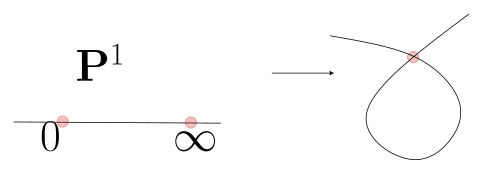Note that $$\operatorname{Pic}(C) = {\mathbf{Z}}\bigoplus {{\mathbf{C}}^{\times}}$$.

For a linear action by a finite group $$G$$, writing $$Z = \operatorname{mProj}R$$ with $$R = k[x_1, \cdots, x_{n}]/\sqrt{\left\langle{f_i}\right\rangle}$$ then $$Z/G = \operatorname{mProj}R^G$$. Such actions can be lifted from $$Z$$ to $${ \left.{{{\mathcal{O}}_{{\mathbf{P}}^n}(1) }} \right|_{{Z}} } = {\mathcal{O}}_Z(1)$$.

## 5.2 Group Varieties

A variety $$G\in{\mathsf{Var}}_{/ {k}}$$ is a group variety if it admits morphisms

• Multiplication $$\mu \in \mathsf{Alg}{\mathsf{Var}}(G \underset{\scriptscriptstyle {k} }{\times} G, G)$$,
• Units: $$e\in \mathsf{Alg}{\mathsf{Var}}(\operatorname{Spec}k, G)$$,
• Inverses: $$i\in \mathsf{Alg}{\mathsf{Var}}(G\to G)$$.

These are required to satisfy some axioms.

Encoding associativity:Encoding $$1a=a$$: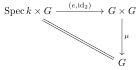Encoding $$aa^{-1}= 1$$:Suppose that $$G = \operatorname{Spec}R$$ is affine, then there are dual notions:

• Comultiplication: $$\mu^*: R\to R\otimes_k R$$.
• Counits: $$e^*: R\to k$$.
• Coinverses: $$i^*: R\to R$$.

The additive group $${\mathbf{G}}_a = \operatorname{Spec}k[x]$$, whose underlying variety is $${\mathbf{A}}^1$$. In coordinates, the group law is written additively as

• $$\mu(x, y) = x+y$$
• $$e = 0$$
• $$i(x) = -x$$

Write $$z=x+y$$, then on the ring side we have

• Comultiplication: \begin{align*} \mu^*: k[z] &\to k[x]\otimes_k k[y] \cong k[x+y] \\ z &\mapsto x\otimes 1 + 1\otimes y &\mapsto x+y .\end{align*}

• Counit: $$e^*: k[x] \to k$$ where $$x\mapsto 0$$

• Coinverse: $$i^*: k[x] \to k[x]$$ where $$x\mapsto -x$$.

The multiplicative group $${\mathbf{G}}_m = \operatorname{Spec}k[x, x^{-1}]$$ whose underlying variety is $${\mathbf{A}}^2\setminus\left\{{0}\right\}$$

The group law is:

• $$\mu(x, y) = xy$$
• $$e = 1$$
• $$i(x) = x^{-1}$$

For rings:

• Comultiplication: \begin{align*} \mu^*: k[z] &\mapsto k[x,x^{-1}]\otimes_k k[y,y^{-1}] \cong k[x^{\pm 1}, y^{\pm 1}] \\ z &\mapsto x\otimes y \mapsto xy .\end{align*}

• Counit: $$e^*: k[x^{\pm 1} ] \to k$$ where $$x\mapsto 1$$.

• Coinverse: $$i^*: k[x^{\pm 1}] \to k[x^{\pm 1}]$$ where $$x\mapsto x^{-1}$$.

Roots of units $$\mu_n = \operatorname{Spec}k[x]/\left\langle{x^n-1}\right\rangle$$. Note that there is a closed embedding $$\mu_n \hookrightarrow{\mathbf{G}}_m$$ since there is a surjection $$\operatorname{Spec}k[x, x^{-1}] \twoheadrightarrow k[x]/\left\langle{x^n-1}\right\rangle$$. Note that in $$\operatorname{ch}k = p$$, this yields a scheme that is not a variety since it is not reduced: one has $$\mu_p = \operatorname{Spec}k[x]/\left\langle{x^p-1}\right\rangle = \operatorname{Spec}k[x]/\left\langle{(x-1)^p}\right\rangle$$ which contains nilpotents. This is the first example of a group scheme which is not a group variety.

The group operations agree with that on $${\mathbf{G}}_m$$, e.g. comultiplication is \begin{align*} \mu^*: k[z]/\left\langle{z^n-1}\right\rangle \to k[x]/\left\langle{x^n-1}\right\rangle\otimes_k k[y]/\left\langle{y^n-1}\right\rangle \cong k[x,y]/\left\langle{x^n-1, y^n-1}\right\rangle .\end{align*} One can similarly define $$\alpha_p = \ker\operatorname{Frob}\hookrightarrow{\mathbf{G}}_m = \operatorname{Spec}k[x]/\left\langle{x^p}\right\rangle$$.

Upcoming: more group varieties and schemes, especially $$\operatorname{GL}_n, {\operatorname{SL}}_n$$, and their actions/coactions.

# 6 Tuesday, September 06

## 6.1 Group varieties

Last time: group varieties. Most of today will work over $${\mathbf{C}}, k\neq { \overline{k} }$$, or $${\mathbf{Z}}$$. There is a correspondence:

Affine varieties Rings and k-algebras
Group varieties/schemes Hopf coalgebras
$$\mu: G\times G\to G$$ $$\mu^*: R\to R\otimes_k R$$
$$e: {\operatorname{pt}}\to G$$ $$e^*: R\to k$$
$$i: G\to G$$ $$i^*: R\to R$$

Recall:

• For $$M, N\in {}_{R}{\mathsf{Mod}}$$, there is a tensor product $$M\otimes_R N$$,
• A morphism $$f\in \mathsf{CRing}(R, S)$$ yields a functor $$f^*: {}_{S}{\mathsf{Mod}}\to ({R}, {S}){\hbox{-}}\mathsf{biMod}$$ given by the base change/scalar extension $$({-})\otimes_R S$$
• If $$S_1, S_2 \in {}_{R} \mathsf{Alg}$$ then $$S_1\otimes_R S_2 \in {}_{R}{\mathsf{Mod}}$$ is in fact a ring, using the product $$(u_1\otimes v_1)\cdot(u_2\otimes v_2) \mathrel{\vcenter{:}}= u_1u_2\otimes v_1 v_2$$.
• For any $$N\in {}_{R}{\mathsf{Mod}}$$, the functor $$({-}) \otimes_R N$$ is right exact, so \begin{align*} A\hookrightarrow B\twoheadrightarrow&C \\ \leadsto \\ A\otimes_R N\to B\otimes_R N \twoheadrightarrow&C\otimes_R N .\end{align*}

If $$M$$ is finitely generated, there is a generator/relation exact sequence $$R{ {}^{ \scriptscriptstyle\oplus^{m} } } \to R{ {}^{ \scriptscriptstyle\oplus^{n} } }\twoheadrightarrow C$$. Tensoring with any $$N\in {}_{R}{\mathsf{Mod}}$$ yields \begin{align*} N { {}^{ \scriptscriptstyle\oplus^{m} } } \to N{ {}^{ \scriptscriptstyle\oplus^{n} } } \twoheadrightarrow C\otimes_R N .\end{align*} In particular, this works for base change $${}_{R}{\mathsf{Mod}}\to {}_{S}{\mathsf{Mod}}$$ – the new module is generated as a module by the same generators but new scalars.

Consider $${\mathbf{C}}\otimes_{\mathbf{R}}{\mathbf{C}}$$, which has a ring structure. Write $${\mathbf{C}}= {\mathbf{R}}[x]/\left\langle{x^2+1}\right\rangle$$, then the base change is \begin{align*} {\mathbf{C}}[x]/\left\langle{x^2+1}\right\rangle = {\mathbf{C}}[x]/\left\langle{x-i}\right\rangle \oplus {\mathbf{C}}[x]/\left\langle{x+i}\right\rangle \cong {\mathbf{C}}\oplus {\mathbf{C}} ,\end{align*} which is a ring with zero divisors and idempotents since $$(1, 0)^2 = (1^2, 0^2) = (1, 0)$$.

For tensor products: same generators, same relations, extend scalars.

Recall:

• $${\mathbf{G}}_m = \operatorname{Spec}k[x^{\pm 1}] \approx {{\mathbf{C}}^{\times}}$$.
• $${\mathbf{G}}_m^n = \operatorname{Spec}k[x_1^{\pm 1}, \cdots, x_n^{\pm 1}] \approx ({{\mathbf{C}}^{\times}})^n$$.
• $${\mathbf{G}}_a = \operatorname{Spec}k[x] \approx ({\mathbf{C}}, +)$$.
• $$\mu_n \subseteq {\mathbf{G}}_m = \operatorname{Spec}k[x]/\left\langle{x^n-1}\right\rangle \approx \left\{{\xi \in {\mathbf{C}}{~\mathrel{\Big\vert}~}\xi^n=1}\right\}$$.
• In characteristic $$p$$, $$\mu_p = \operatorname{Spec}k[x]/\left\langle{x^p-1}\right\rangle = \operatorname{Spec}k[x]/\left\langle{x-1}\right\rangle^p$$.

Of using the tensor product slogan: identifying the map \begin{align*} { k[z] \over \left\langle{z^n-1}\right\rangle} \to {k[x] \over \left\langle{x^n-1}\right\rangle} \otimes_k {k[y]\over \left\langle{y^n-1}\right\rangle } \cong {k[x,y]\over \left\langle{x^n-1, y^n-1}\right\rangle } ,\end{align*} realizing this as $$z\mapsto x\otimes y\mapsto xy$$, checking that $$z^n=1 \implies (xy)^n = 1$$.

If $$G$$ is an arbitrary finite group it can be made into an affine algebraic group variety over $$k$$. Give the underlying set of $$G = {\textstyle\coprod}_{g\in G}\left\{{{\operatorname{pt}}}\right\}$$ the discrete topology to get an algebraic variety. To get the algebraic group structure, note that any map of finite sets is algebraic. Define a ring $$R \mathrel{\vcenter{:}}=\bigoplus _{g\in G} k$$, and a comultiplication as follows: note that \begin{align*} R\otimes_k R \cong \bigoplus _{(a, b) \in G\times G } k e_{a,b} \end{align*} where the $$e_{a, b}$$ just tracks which summand we’re in. So define \begin{align*} R &\to R\otimes_k R \\ e_g &\mapsto \sum_{ab=g} e_{a, b} .\end{align*}

Note that we could have let $${\operatorname{pt}}= \operatorname{Spec}k$$. E.g. $$C_p \neq \mu_p$$ but are Cartier dual. However, the ring $$k[x]/\left\langle{x-1}\right\rangle^p$$ is much easier to understand than the $$R\otimes_k R$$ from above, even for very small groups like $$C_2$$.

Recall $$\operatorname{GL}_n \subseteq {\mathbf{A}}^{n^2}$$ is the open subspace which is the complement of $$V(\operatorname{det})$$, so a principal open subset. The ring is $$k[x_{ij}, 1/\operatorname{det}]$$ for $$1\leq i,j\leq k$$, which is obtained by localizing at the determinant. Thus we can embed it as a closed subset in $${\mathbf{A}}^{n^2+1}$$ using $$V(y\operatorname{det}(x_{ij}) = 1)$$, i.e. introducing a new free variable for $$1/\operatorname{det}$$ and ensuring it’s nonzero.

An affine algebraic group is a closed subgroup $$G$$ of $$\operatorname{GL}_n$$, and the coordinate ring $$R_G$$ is a quotient of $$R_{\operatorname{GL}_n}$$.

For $${\operatorname{SL}}_n$$, the ring is $$k[x_{ij}]/\left\langle{\operatorname{det}- 1}\right\rangle$$, and define $$\operatorname{PGL}_n$$ as $${\operatorname{SL}}_n/\mu_n$$ or $$\operatorname{GL}_n/{\mathbf{G}}_m$$. Although it’s not obvious, these are affine – for $$\operatorname{PGL}_n$$, the ring is the $$\mu_n$$ invariants of the coordinate ring of $${\operatorname{SL}}_n$$, so one gets the ring of polynomials in $$R_{{\operatorname{SL}}_n}$$ whose powers are multiples of $$n$$.

## 6.2 Algebraic group actions

An action of an algebraic group $$G$$ on a variety (or scheme) $$X$$ is a map $$G \underset{\scriptscriptstyle {k} }{\times}X \xrightarrow{a} X$$ satisfying the usual axioms encoded in commuting diagrams:

• $$(gh).x = g.(h.x)$$:• $$1.x = x$$ for all $$x$$:Note that one can now reverse these diagrams to get the coaction on rings $$a^*: A\to R\otimes_k A$$.

Let $$\mu_n \curvearrowright{\mathbf{A}}^2$$ by $$\xi.(x,y) \mathrel{\vcenter{:}}=(\xi x, \xi^k y)$$, then the coaction is \begin{align*} k[x,y] &\to {k[x,y,\xi] \over \left\langle{\xi^n-1}\right\rangle } = {k[\xi] \over \xi^n-1}[x, y] \\ x &\mapsto \xi x \\ y &\mapsto \xi^k y .\end{align*}

Check that this satisfies the axioms for a coaction.

Let $$G\in {\mathsf{Grp}}{\mathsf{Var}}_{/ {k}}, X\in { \mathsf{Vect}}_{/ {k}}$$, then a linear coaction is a homomorphism $$V \xrightarrow{a^*} R\otimes_k V$$ satisfying the duals of the axioms above.

If $$V = kx \oplus ky$$ then $$V\to R\otimes_k V = Rx \oplus Ry$$.

There is a coaction on $$A = \operatorname{Sym}^* V = k \oplus V \oplus \operatorname{Sym}^2 V \oplus \cdots$$, where $$V = \left\langle{x,y}\right\rangle, \operatorname{Sym}^2 V = \left\langle{x^2, xy, y^2}\right\rangle$$. This is the same as an action $$G\curvearrowright{\mathbf{A}}^N$$ for some $$N$$ acting on an affine space.

# 7 Thursday, September 08

## 7.1 Diagonalizable groups

Last time: coactions on vector spaces $$a^*: V\to R\otimes_k V$$ where $$R = k[G]$$ is the ring of regular functions on an algebraic group $$G$$. Thinking of $$V {}^{ \vee }\cong k^n \cong {\mathbf{A}}^n_{/ {k}} = \operatorname{Spec}\operatorname{Sym}^*V$$ as the ring of regular functions, we get a map $$\operatorname{Sym}^*(V) \to R\otimes_k \operatorname{Sym}^*(V)$$.

A vector $$v\in V$$ is invariant if $$a^*(v) = 1\otimes v$$.

Every algebraic coaction is locally finite, i.e. every $$v\in V$$ is contained in a finite-dimensional invariant vector subspace.

Check that $$v\mapsto \sum a_i \otimes v_i$$ where $$v\in \left\langle{v_i}\right\rangle$$ and use $$a.(b.v) = (ab).v$$.

Let $$A$$ be a finitely-generated abelian group and let $$G\mathrel{\vcenter{:}}=\widehat{A}$$ be its Cartier dual. Then $$R_G = k[A] \mathrel{\vcenter{:}}=\left\{{\sum c_a e^a {~\mathrel{\Big\vert}~}c_a\in k, e^{a}e^{b} = e^{a+b}}\right\}$$ is a commutative ring and in fact a finitely-generated algebra. For $$k$$ a general ring, this yields a scheme, and in fact it has the structure of a group scheme: \begin{align*} e^*: R_G &\to R_G \otimes_k R_G \\ e^c &\mapsto \sum_{a+b=c} e^a\otimes e^b \mathrel{\vcenter{:}}=\sum_{a+b=c}e^{(a, b)} \\ \\ u^*: R_G &\to k \\ e^a &\mapsto 1 \\ \\ i^*: R_G &\to R_g \\ e^a &\mapsto e^{-a} \\ \\ .\end{align*} Note that $$A \cong {\mathbf{Z}}^r \bigoplus {\mathbf{Z}}/n_i{\mathbf{Z}}$$, so all diagonalizable groups are of the form $$\widehat{A} = {\mathbf{G}}_m^r \bigoplus \mu_{n_i}$$.

• $$A={\mathbf{Z}}$$ yields $$\widehat{A} = {\mathbf{G}}_m = \operatorname{Spec}k[{\mathbf{Z}}]$$.
• $$A = C_n$$ yields $$\widehat{A} = \mu_n = \operatorname{Spec}k[C_n]$$ which has nilpotents.
• $$A = {\mathbf{Z}}^r$$ yields $$\widehat{A} = \operatorname{Spec}k[{\mathbf{Z}}^r]$$, and choosing a basis for $${\mathbf{Z}}^r$$ yields an isomorphism with $$\operatorname{Spec}k[x_1^{\pm 1}, \cdots, x_r^{\pm 1}]$$.

An algebraic coaction $$\widehat{A}\curvearrowright V$$ yields a grading $$V = \bigoplus _{a\in A} V_a$$. Thus a $${\mathbf{G}}_m$$ action is a $${\mathbf{Z}}{\hbox{-}}$$grading, and a $$\mu_n$$ action is a $$C_n{\hbox{-}}$$grading. This works for $$k$$ any ring.

Check that $$V \xrightarrow{a^*} V\otimes k[A]$$ by $$v\mapsto \sum_{a\in A} e^a v_a$$. This is a finite sum, so there are only finitely many nonzero $$v_a$$ appearing in this sum. We need to show

• $$v = \sum v_a$$,
• $$v_a\mapsto (v_a\in V_a, 0\in V_{b\neq a})$$,
• $$v_a \neq \sum_{b\neq a} v_b$$.

For the first, compose $$V \xrightarrow{e^*} V\otimes R \xrightarrow{u^*} V$$ by $$v\mapsto \sum e^a v_a \mapsto \sum v_a$$ and this must equal $$v$$ by the axioms. For the second, first using $$g(hv)$$ to get $$v\mapsto \sum e^a v_a \mapsto (v_a)_b e^a \otimes e^b$$, and $$(gh)v$$ to get $$v\mapsto \sum e^av_a \mapsto \sum_{b+c=a} v_a e_b \otimes e^c$$. These must be equal, so the coefficients must be equal.

Check this – show that the last equality is equivalent to being a direct sum.

## 7.2 Invariants

Let $$G\in \mathsf{Alg}{\mathsf{Grp}}{\mathsf{Var}}_{/ {k}}$$ and suppose $$G\curvearrowright V$$ is a $$G{\hbox{-}}$$representation, i.e. a coaction $$V\to R\otimes V$$. Define the invariant subspace $$V^G \mathrel{\vcenter{:}}=\left\{{v\in V{~\mathrel{\Big\vert}~}a^*(v) = 1\otimes v}\right\} \subseteq V$$; $$G$$ is linearly reductive iff for any $$V\twoheadrightarrow W$$ of $$G{\hbox{-}}$$representations induces $$V^G \twoheadrightarrow W^G$$.

One can equivalently require $$V,W$$ to be arbitrary or just finite-dimensional.

If $$G$$ is a finite group variety and $$\operatorname{ch}k \notdivides {\sharp}G$$, then $$G$$ is linearly reductive.

Use the Reynolds operator $$V \xrightarrow[]{R} { \mathrel{\mkern-16mu}\rightarrow }\, V^G$$ which sections the inclusion $$V^G \hookrightarrow V$$, so $$R \circ i = \operatorname{id}_{V^G}$$, where $$R(v) = ({\sharp}G)^{-1}\sum_{g\in G} g(v)$$.

Any diagonalizable group is linearly reductive.

Writing $$V = \bigoplus _{a\in A} V_a$$, then $$V^G = V_0$$ and projecting onto the $$a=0$$ summand yields a surjection.

Over $$\operatorname{ch}{ \mathbf{F} }= p$$, the only linearly reductive groups are either finite or diagonalizable.

Over $${\mathbf{C}}$$, the linearly reductive groups are precisely

• $${\mathbf{G}}_m^r$$
• Semisimple groups of types $$A ({\operatorname{SL}}_n),B,C,D ({\operatorname{SO}}_n, {\operatorname{Sp}}_n),E_{6,7,8},F_4, G_2$$.
• $$T\times G/H$$ for $$G$$ semisimple and $$H$$ a finite central subgroup.

This includes $$\operatorname{GL}_n = {\operatorname{SL}}_n \times {{\mathbf{C}}^{\times}}/ H$$ and $$\operatorname{PGL}_n = {\operatorname{SL}}_n/\mu_n$$.

Later: invariants of finitely generated for linearly reductive are again finitely generated. Note that invariants can be finitely-generated even when the group is not.

# 8 Tuesday, September 13

Suppose $$G$$ is a linearly reductive group and $$G\curvearrowright R$$ a finitely generated ring (or $$k{\hbox{-}}$$algebra). Then the subring of invariants $$R^G$$ is finitely generated.

See proof in Mukai, due to Hilbert.

Suppose $$G\curvearrowright S \mathrel{\vcenter{:}}= k[x_0, \cdots, x_n]$$ linearly and preserves the grading. Then $$S^G$$ is finitely generated.

Since $$G$$ preserves polynomials of degree $$d$$, the ring $$S^G$$ is graded and decomposes as $$S^G = \bigoplus _{e\geq 0} S^G \cap S_e$$. Let $$S_+^G$$ be the elements of positive degree and write $$S^G = k \oplus S_+^G$$. Writing $$J = \left\langle{S_+^G}\right\rangle {~\trianglelefteq~}S$$ for the ideal it generates, since $$S$$ is Noetherian then we can write $$J = \left\langle{f_1,\cdots, f_N}\right\rangle$$. These can be chosen to be homogeneous by choosing any homogeneous polynomial, stopping if that generates the ring, and otherwise continuing by picking $$f_i$$ in the complement to construct an ascending chain of ideals.

\begin{align*} S^G\in {}_{k} \mathsf{Alg} .\end{align*}

Take $$f_i$$ such that $$\deg(f_i) > 0$$. There is a surjective morphism $$S{ {}^{ \scriptscriptstyle\oplus^{N} } } \twoheadrightarrow J$$ of $$S{\hbox{-}}$$modules. Since $$J^G \subseteq S^G$$, this yields a surjection $$(S^G){ {}^{ \scriptscriptstyle\oplus^{N} } } \twoheadrightarrow J^G$$ of $$G{\hbox{-}}$$modules. If $$f\in J^G$$ then write $$f = \sum_{i=1}^N h_i f_i$$ with $$h_i\in S^G$$ and $$\deg h_i < \deg f_i$$. Finish by induction.

This yields a surjection $$S = k[x_0,\cdots, x_n] \twoheadrightarrow R$$